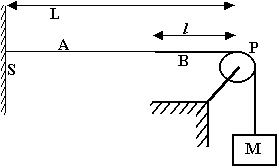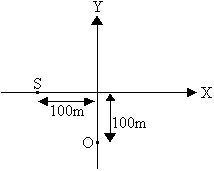# IIT Physics Sample Paper 2

### Waves and Sound

1.Three metal rods are located relative to each other as shown in the figure where L1 + L2 = L3. Values of density and Youngs modulus for the three materials are:
d1 = 2.7 ´ 103 kgm-3, Y1 = 7 ´ 1010 Nm-2
d2 = 11.3 ´ 103 kgm-3, Y2 = 1.6 ´ 1010 Nm-2
d3 = 8.8 ´ 103 kgm-3, Y3 = 11 ´ 1010 Nm-2
If L3 = 1.5 m, what must be the ratio L1/L2 be if a sound wave is to travel the length of the rods 1 and 2 in the same time required to travel the length of rod 3? If the frequency of the source is 4000hz, determine the phase difference between the wave traveling along rods 1 and 2 and the one traveling along rod 3.
2.Two speakers are arranged as shown. Assume that point O is 12m along the center line and the speakers are separated by a distance of 1.5m. As the listener moves toward point P from point O, a series of alternating minima and maxima are encountered. The distance between the first minimum and the next maximum is 0.4m. Using 340 m/s as speed of sound in air, determine the frequency of the speakers.
3.A triangular wave pulse on a string with a tension F is moving in the positive x direction with a velocity of 50 m/s. The linear mass density is m = 0.080 kg/m. The shape of the pulse is as shown. Show that the energy of the pulse is E=2Fh2/L. Find the value of E.
4. At t = 0, a transverse wave pulse in a wire is described by the function y=6/(x2-3) where x and y are in m. Write the function y(x,t) that describes this wave traveling in the positive x direction with a speed of 4.5m/s.

5.Consider the shown arrangement in which a thin rope A with a linear density mA is connected to a thick rope B with linear mass density mB. The thick rope passes over a pulley and is connected to a heavy block of mass M. The separation of fixed support S from a pulley is L. A stationary wave is setup in this composite string such that the joint remains a node. Given that mb = 4 kg/m, ma = 0.4 kg/m, M = 1 kg and L = 4l = 1 m. Under these conditions, find:
1. The least possible frequency of vibration
2. The total energy of vibration if the amplitude for both the string is A = 1 mm and the string vibrates at the frequency obtained in (a).

6.At t = 0, a source of sonic oscillations S and an observer O start moving along positive x and positive y axis respectively with velocities 5 m/s and 10 m/s. The figure gives their position at t = 0. The frequency of sonic oscillations at source is 100 Hz. Obtain the frequency of signals received by the observer right at the moment when source crosses origin.
7. A rope of length l and mass m hangs from the ceiling. If a wave of frequency n propagates through the rope, find the phase difference between the top and the bottom of the rope.
8. A uniform rope of mass m and length l is whirled in a horizontal circle about one of its ends with an angular velocity w. Find the time taken by a transverse pulse to travel from one end of the rope to the other. Ignore g.
9. A wire when stretched by a weight of a solid gives a frequency ¦. When the solid is immersed in water it gives a frequency of ¦/2 and when immersed in a liquid of specific gravity r, it gives a frequency 3¦/4. Find the specific gravity of the liquid.
10. A string 120 cm in length sustains a standing wave with the points of the string at which the displacement amplitude is equal to 3.5 mm being separated by 15 cm. Find the maximum displacement amplitude and the overtone to which the oscillations belong.
11. A string of mass m is fixed at both ends. The fundamental tone oscillations are excited with angular frequency w and maximum displacement amplitude amax. Find
1. The maximum kinetic energy of the string.
2. The mean kinetic energy of the string averaged over one oscillation period.
12. A metal wire of length 1 m mass 0.1 kg and uniform cross-sectional 10-6 m2 is rigidly fixed at both ends at zero tension. The temperature of the wire is then lowered by 40°C and transverse waves are setup by plucking the wire in the middle.
1. Calculate the frequency of the vibration (fo) of the wire.
2. Compare this frequency with the fundamental frequency (fo) emitted by the wire when set into longitudinal vibrations before lowering the temperature. (express as ratio)
Given Linear expnsivity of metal = 1.21 x 10-5/°C
Youngs modulus = 2 x 1011 N/m2.IIT JEE - HomeWebsites of IITJEE SyllabusIIT Question PapersBooks For IITIIT JEE 2008IIT JEE 2007IIT JEE 2006IIT JEE 2005IIT CoursesSeats ReservationEligibility CriteriaAnalysis of IIT JEEIIT AlumniIIT JEE TipsIIT Articles